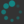1. Show that $X$ is contractible if and only if every map $f\colon Y\to X,$ for arbitrary $Y,$ is nullhomotopic. Dually, show that $X$ is contractible if and only if every map $f\colon X\to Y,$ for arbitrary $Y,$ is nullhomotopic.